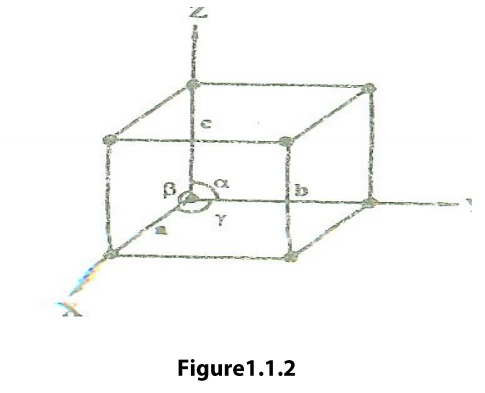Home | | Engineering Physics I | Lattice Parameters or Unit Cell Parameters

# Lattice Parameters or Unit Cell Parameters

The lines drawn parallel to the lines of intersection of any three faces of the Unit Cell which do not lie in the same plane are called Crystallographic Axis.

Unit Cell is defined as the smallest geometric figure or pattern, the repetition of which gives the actual crystal structure. This is also defined as undamental elementary pattern o minimum of atoms or molecules or groups of molecules, which represents total characteristics of the material or the crystal.Lattice Parameters or Unit Cell ParametersThe lines drawn parallel to the lines of intersection of any three faces of the Unit Cell which do not lie in the same plane are called Crystallographic Axis.

The intercepts a,b, c (i.e., the distance between the two lattices points ) are nothing but the edges of the unit cell, which defines the dimensions of unit cell. These intercepts are known as its primitives or characteristic intercepts on the axes.

These three quantities a, b, c is also called the fundamental translational vectors or axial lengths. The angles (a,b), (b,c) and (c,a) are denoted by α,ẞ,γ respectively. These three angles (α,ẞ,γ) are called Interfacial angle.

But the intercept (a,b,c) and the interracial angle (α,ẞ,γ) constitutes the lattice parameters o the unit cell.

The actual size o the unit cell can be determined by knowing the values o intercepts and interracial angles.

Primitive Cells.

A Primitive Cellsis the simplest type of the unit cell, which contains only one lattice point per unit cell. (Contains lattice points at its corner only)

Example: Simple Cubic

Non Primitive Cell

A Non Primitive Cell is one, which contains more than one lattice point per unit cell.

Example: BCC and FCC contain more than one unit cell.

If the number of lattice points per unit cell is two (BCC), three and four(FCC), then the unit cell is called doubly primitive, triply primitive and Quadruple Primitive respectively.

Study Material, Lecturing Notes, Assignment, Reference, Wiki description explanation, brief detail
Physics : Crystal Physics : Lattice Parameters or Unit Cell Parameters |